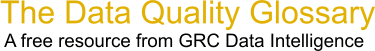Choose Index below for a list of all words and phrases defined in this glossary.

# R-Square

index | Index

## R-Square - definitions

R-Square - A mathematical term describing how much variation is being explained by the X.

Rsq = 1 - SS(regression)/SS(total), Assuming "SS" = Sum Squared error, and that "SS(total)" means the variance in the data. This should be obvious, as R-squared approaches unity as a regression approaches a perfect fit.(i.e., Rsq = 1 - sum/sum

The R-squared value is the fraction of the variance (not 'variation') in the data that is explained by a regression.

[Category=Data Quality ]

Source: iSixSigma, 19 February 2011 11:46:58, http://www.isixsigma.com/index.php?option=com_glossaryThese advertisers support this free service

R-squared - [statistics] A statistic computed by the regression equation to quantify model performance. The value of R-squared ranges from 0 to 100 percent. If a model fits the observed dependent variable values perfectly, the R-squared value is 1.0, although this is highly unlikely. An R-squared value like 0.49, for example, is far more likely, and means that the model explains 49% of the variation in the dependent variable.

[Category=Geospatial ]

Source: esri, 08 August 2012 08:49:49, http://support.esri.com/en/knowledgebase/GISDictionary/term/abbreviationData Quality Glossary.  A free resource from GRC Data Intelligence. For comments, questions or feedback: dqglossary@grcdi.nl